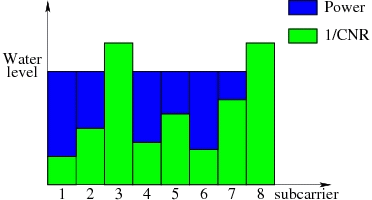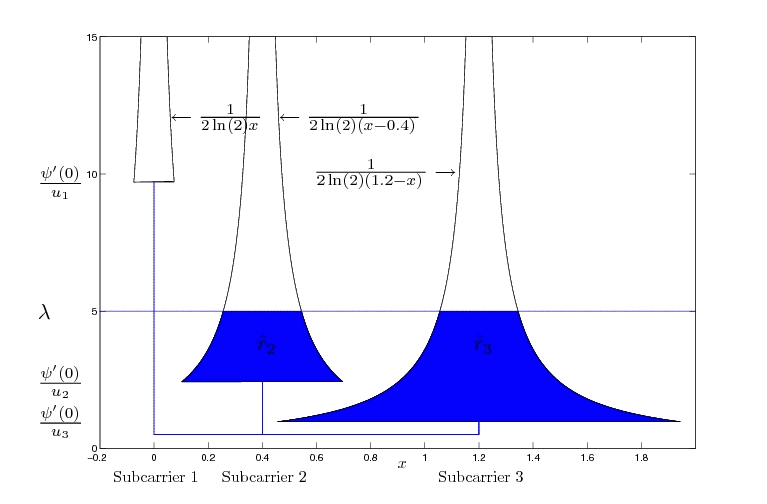# Optimum Resource AllocationIn an orthogonal frequency-division multiplexing (OFDM) system, the frequency band is split into multiple independent resource blocks that can be modeled as non-interfering flat narrowband channels. In OFDMA (OFDM Multiple Access) systems, multiple users are served simultaneously by assigning these blocks or subcarriers to users in a way that each subcarrier is occupied by at most one user. Adaptive coding and modulation techniques allow users to adapt their data transmission scheme to the wireless channel conditions on a per-subcarrier basis, maximizing frequency diversity gain. Energy efficiency and high data rates are important design principles for future wireless systems based on OFDMA. Consequently, there are basically the following aproaches:

• Rate adaptive (RA) optimization, which maximizes the overall rate given a fixed power budget.
• Margin adaptive (MA) optimization, where the transmitted power is minimized while fulfilling certain user rate demands.
• A mixture of RA and MA optimization.

The combinatorial nature of these problems is computationally prohibitive, such that the development of approximation techniques and algorithms plays an important practical role. However, by combining results from integer, convex, and nonconvex optimization theory, performance estimates and bounds for these approaches can be derived.

As intermediate step the discrete rate-power step function is often approximated by a continuous convex function in order to apply algorithms from convex optimization for efficient evaluation.

The resource allocation algorithms usually rely on the fact that a feasible solution exists. Hence, admission control on one hand should ensure that the resource allocation is able to find a solution and on the other hand should refuse as few users as possible. Altogether admission control is a challenging task, particularly, as it should take into account the nearby future.